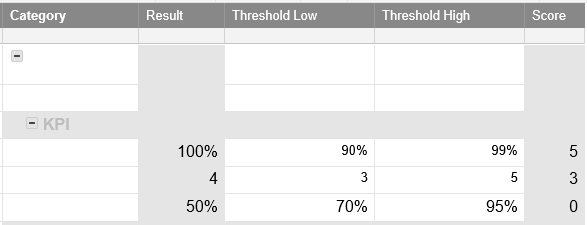# Need Help with formula: One of 3 values based on a high and low threshold

Trying to set up a formula (IF?) where the value of a cell is either 0, 3 or 5 determined by the value of a result and if it is below a minimum threshold, in the middle or above a high threshold.  For the attached example, the first KPI result is 100% so it receives 5, second result is 4 falls in the middle so receives 3, third result is 50% is below minimum so it receives 0Tags:

• Try this with the assumption that the first KRI result is in row 4. If you build this formula out in the score column that part will build out automatically. Hope this helps!

=IF(ISBLANK([Result]4), "", IF([Result]4 < [Threshold Low]4, 0, IF([Result]4 > [Threshold High]4, 5, 3)))

• Thanks so much, worked perfectly!  I just realized that I have a few KPI's that are inverse.  How do I reverse so that Less than a certain number gets the score of 5 etc.

• I answered my own question.  reversed the >< in the formula.  Thanks again!

• Glad I could help and that you sorted out the issue!

## Help Article Resources

Want to practice working with formulas directly in Smartsheet?

Check out the Formula Handbook template!